# 微波加热金属导体的仿真研究Simulation Study on Microwave Heating of Metallic Conductor

DOI: 10.12677/MOS.2021.101007, PDF, HTML, XML, 下载: 82  浏览: 141  科研立项经费支持

Abstract: Based on finite element software COMSOL Multiphysics, a bidirectional model of electromagnetic field and thermal conductivity of copper electrode that placed in the resonant cavity is established. The optimal position that has the highest thermal efficiency is confirmed by parametric sweep of coordinates based on analyzing its heat source. In addition, the relation between electrode’s surface roughness and its temperature is researched. It is found that the temperature increases drastically with the surface roughness of 0~10 μm. It becomes relatively stable at 2250˚C~2264˚C of 10~50 μm, which is able to stimulate to generate in-liquid plasma.

1. 引言

Satoshi Horikoshi，Nick Serpone等人  提出一套利用微波加热金属电极(Electrode)产生等离子体的反应装置，如图1所示。在该装置中微波发生器(MW generator)产生微波，波导管(Waveguide)将微波导入腔体，微波在腔体中连续发射，最终部分微波在电极表面产生感应电流引发焦耳热，电极顶端产生2000℃以上的高温从而激发等离子体产生。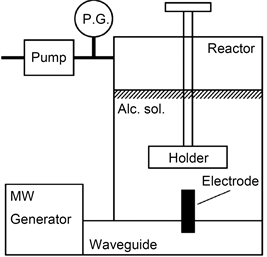Figure 1. Apparatus of microwave heating of metallic electrode to generate plasma 

Ismail Rahim等人  曾利用类似的装置在2.45 GHz微波和磁控管功率为700 W的条件下激发金属表面产生高温，激发可燃冰产生等离子体获取氢能。

2. 加热装置和模型参数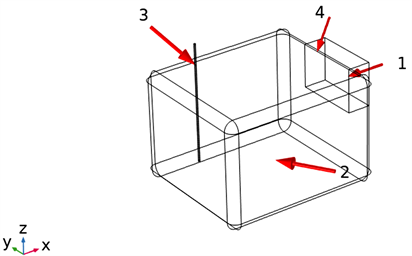1.MW (f = 2.45 GHz)；2.谐振腔；3.铜电极；4.波导(P = 500 W)

Figure 2. Schematic diagram of microwave heating apparatus in simulation

3. 条件假设与边界条件

1) ${\epsilon }_{th}=\alpha \left(T-{T}_{ref}\right)$ (2.1)

2) $-n\cdot q=0$ (2.2)

3) $n×E=0$ (2.3)

$\nabla ×{\mu }_{r}^{-1}\left(\nabla ×E\right)-{k}_{0}^{2}\left({\epsilon }_{r}\left(T\right){\epsilon }_{0}-\frac{j\sigma \left(T\right)}{\omega }\right)E=0$ (2.4)

${k}_{0}=\omega \sqrt{{\mu }_{0}{\epsilon }_{0}}$ (2.5)

${\epsilon }_{r}\left(T\right)={\epsilon }_{\infty }+\frac{{\epsilon }_{s}-{\epsilon }_{\infty }}{1+j2\pi f\tau }$ (2.6)

$\tau$ 为弛豫时间。其中， ${\epsilon }_{\infty }=5.5$${\epsilon }_{s}=9×{10}^{-4}{T}^{2}-0.403T+88.1$$\tau =9.47×{10}^{-10}/{\left(T+22.05\right)}^{1.23}\text{s}$

${J}_{si}=\frac{{E}_{ti}}{{Z}_{s}},i=\text{up},\text{down}$ (2.7)

${Z}_{s}=\sqrt{\frac{{\mu }_{0}{\mu }_{r}}{{\epsilon }_{0}{\epsilon }_{r}\left(T\right)-j\sigma \left(T\right)/\omega }}$ (2.8)

${Z}_{rough}={Z}_{smooth}\cdot \left[1+\frac{2}{\pi }{\mathrm{tan}}^{-1}\left(1.4{\left(\frac{{\text{Δ}}_{RMS}}{\delta }\right)}^{2}\right)\right]$ (2.9)

$\rho {C}_{p}\frac{\partial T}{\partial t}=\nabla \cdot \left(k\nabla T\right)+Q$ (2.10)

4. 铜电极热源及其温度场

${Q}_{e}={Q}_{rh}+{Q}_{ml}$ (3.1)

${Q}_{rh}=\frac{1}{2}Re\left(J\cdot {E}^{*}\right)$ (3.2)

${Q}_{ml}=\frac{1}{2}Re\left(i\omega B\cdot {H}^{*}\right)$ (3.3)

${Q}_{sh}={Q}_{srh}+{Q}_{sml}$ (3.4)

${Q}_{srh}=\frac{\omega {\epsilon }_{0}{{\epsilon }^{″}}_{r}\sum {|{E}_{t,i}|}^{2}}{4{k}^{″}}$ (3.5)

${Q}_{sml}=\frac{\omega {\mu }_{0}{{\mu }^{″}}_{r}\sum {|\frac{{E}_{t,i}}{{Z}_{c}}|}^{2}}{4{k}^{\text{'}\text{'}}}$ (3.6)

${{\epsilon }^{″}}_{r}$${{\mu }^{″}}_{r}$ 分别代表材料边界的相对介电常数虚部及相对磁导率虚部。 ${{\epsilon }^{″}}_{r}$ 为外加磁场作用下，介质电偶矩重排引起损耗的量度， ${{\mu }^{″}}_{r}$ 为外加磁场作用下，介质磁偶矩重排引起损耗的量度，二者承担着电磁波吸波能力，它们引起能量的损耗 。铜属于非铁磁性材料， ${{\mu }^{″}}_{r}$ 为0，则 ${Q}_{sml}$ 亦为0，故 ${Q}_{sh}={Q}_{srh}$。由式(3.5)可见电场强度对Qsh的影响更大。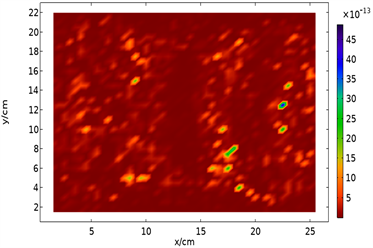(a) Qe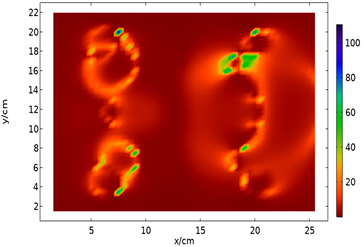(b) Qsh

Figure 3. Numerical distribution of heat source in xy plane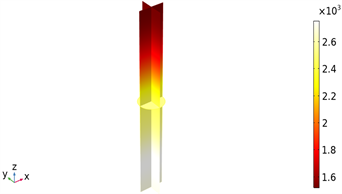(a) Qe + Qsh的温度场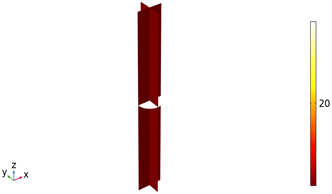(b) Qe的温度场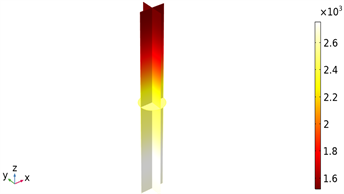(c) Qsh的温度场

Figure 4. Temperature distribution of copper electrode using Qe + Qsh, Qe, Qsh as heat source respectively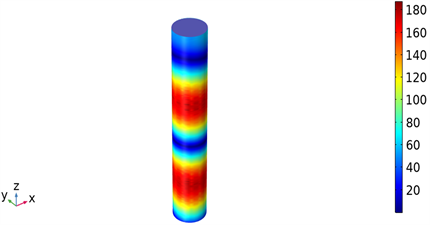Figure 5. Distribution of $|{E}_{t,i}|$Figure 6. Temperature change of copper electrode along z direction

5. 加热位置对平均温度和|S11|的影响

5.1. 平均温度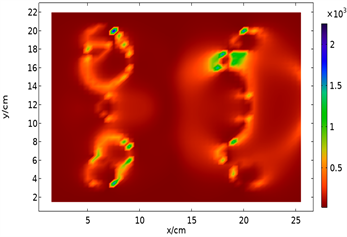Figure 7. Numerical distribution of mean temperature in xy plane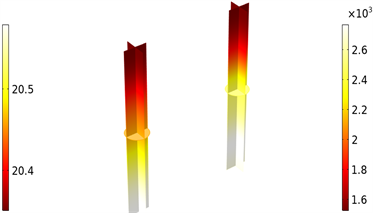Figure 8. Temperature field of copper electrode at (7.5, 20) and (19, 1.5)

5.2. $|{S}_{11}|$

S参数(即散射参数)描述了电磁波在滤波器、天线、波导转换和传输线等设备的不同端口上的传输和反射。它来源于传输线理论，由发射和反射电压波定义。如果存在两个端口，并分别以数字1和2表示，端口1是入口，则S11为端口1处的电压反射系数，S21为端口1至端口2处的电压传输系数。或者说S11是反射波的S参数，S21是透射波的S参数。为了方便，S11使用以下关系式表出 ：

${S}_{11dB}=20{\mathrm{log}}_{10}|{S}_{11}|$ (4.2.1)Figure 9. Numerical distribution of $|{S}_{11}|$ in xy plane

5.3. 平均温度与 $|{S}_{11}|$ 、Qsh的关系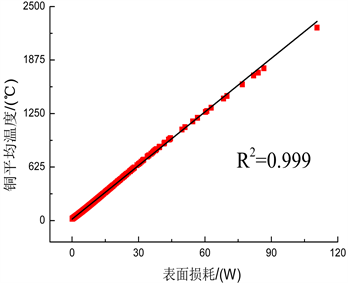(a)(b)

Figure 10. Scatter diagram of mean temperature related to Qsh and $|{S}_{11}|$ respectively

6. 表面粗糙度对平均温度和 $|{S}_{11}|$ 的影响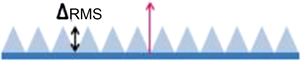Figure 11. Diagram of surface roughness (upside)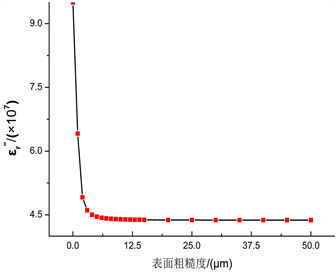(a)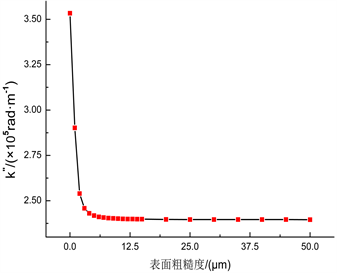(b)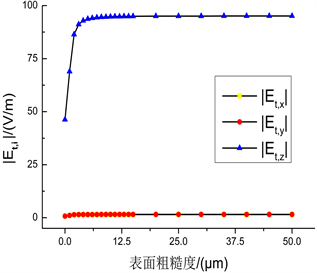(c)

Figure 12. Relations between ${{\epsilon }^{″}}_{r}$, ${k}^{″}$, $|{E}_{t,i}|$ and surface roughness respectively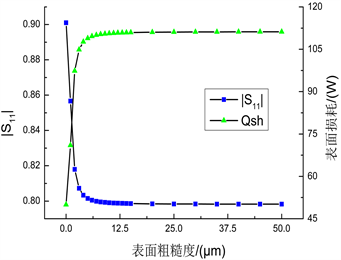(a)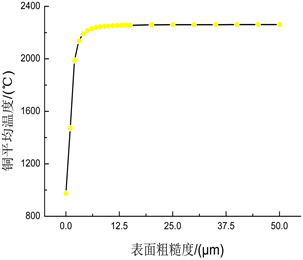(b)

Figure 13. Relations between Qsh, $|{S}_{11}|$, mean temperature and surface roughness respectively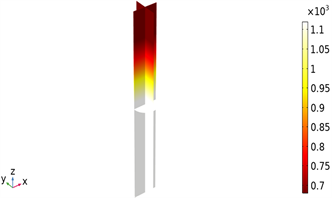(a) 0 μm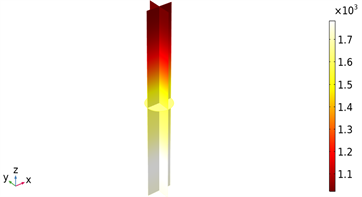(b) 1 μm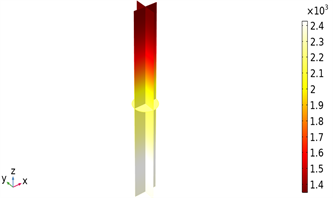(c) 2 μm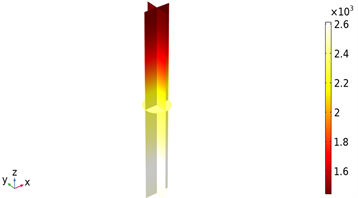(d) 3 μm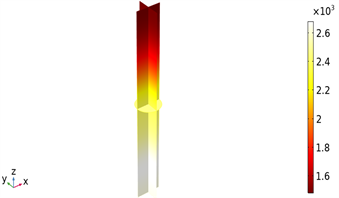(e) 4 μm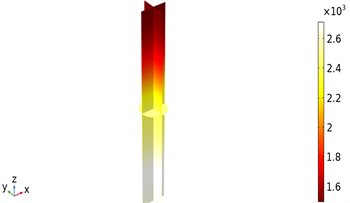(f) 5 μm

Figure 14. Temperature field of copper electrode with surface roughness of 0, 1, 2, 3, 4, 5 μm

7. 结论

NOTES

*通讯作者。

  王青. 微波诱导金属放电催化转化生物质焦油的研究[D]: [硕士学位论文]. 济南: 山东大学, 2018.  刘振. 微波诱导热解电子废弃物过程中金属放电热效应研究[D]: [硕士学位论文]. 济南: 山东大学, 2012.  周玉立. 微波诱导金属放电强化生物质焦油裂解试验与机理研究[D]: [博士学位论文]. 济南: 山东大学, 2018.  Horikoshi, S. and Serpone, N. (2017) In-Liquid Plasma: A Novel Tool in the Fabrication of Nanomaterials and in the Treatment of Wastewaters. RSC Advances 7, 47196-47218. https://doi.org/10.1039/C7RA09600C  Rahim, I., Nomura, S., Mukasa, S., et al. (2015) Decomposition of Methane Hydrate for Hydrogen Production Using Microwave and Radio Frequency In-Liquid Plasma Methods. Applied Thermal Engineering, 90, 120-126. https://doi.org/10.1016/j.applthermaleng.2015.06.074  Zhu, H., He, J., Hong, T., et al. (2018) A Rotary Radi-ation Structure for Microwave Heating Uniformity Improvement. Applied Thermal Engineering, 141, 648-658. https://doi.org/10.1016/j.applthermaleng.2018.05.122  周明长, 李少甫. 基于数值仿真的多馈微波加热温度控制系统[J]. 微波学报, 2019(5): 92-96.  COMSOL (2018) COMSOL Multiphysics User’s Guide, Version: 5.4. CM021001. COMSOL, Stockholm.  Hammerstad, E. and Jensen, O. (1980) Accurate Models for Microstrip Computer-Aided Design. International Microwave Symposium, Washington, DC, USA, May 1980, 407-409.  叶菁华, 于雨田, 洪涛, 等. 微波多模腔金属边界移动对加热的影响研究[J]. 四川大学学报(自然科学版), 2018, 55(1): 87-94.